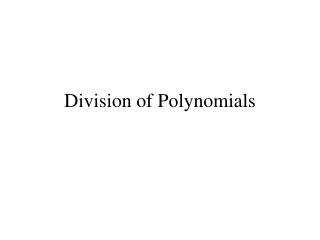DownloadDownload PresentationDivision of Polynomials

Division of Polynomials

Télécharger la présentationDivision of Polynomials

- - - - - - - - - - - - - - - - - - - - - - - - - - - E N D - - - - - - - - - - - - - - - - - - - - - - - - - - -
Presentation Transcript

1. Division of Polynomials

2. Division by a Monomial When dividing monomials with Exponential expressions, use the Quotient Rule of Exponents: if x = 0

3. When subtracting exponents, you might get a zero or a negative exponent. Thus, remember to use the Zero - Exponential Rule and the Negative Exponential Rule: x0 = 1, If x = 0

4. Divide coefficients Example 1: Divide terms with the same bases = - 5 xy 4 xhasdegree 1; 1 can be omitted

5. Example 2:

6. Dividing a Polynomial by a Monomial If a polynomial of more terms is divided by a monomial, divide each term of the polynomial by the monomial. Remember!Always think about which term is positive or negative

7. For example: Remember to divide 3y into every term = y4 + 4y3 – 6y

8. Dividing a Polynomial by a Binomial Example: (x3 + 4x2 + 2x – 6)  (x + 2) Divisor Dividend We are to find the Quotient to which, by Division fact, when it multiplies the divisor, the product must be equal to the dividend.

9. The solution to this question can be found by Long Division, and it usually written in the form: Dividend Divisor Remainder Divisor = Quotient + Long Division can be checked by the following fact: Dividend = Quotient  Divisor + Remainder

10. Long Division Algorithm When the divisor has more than one term, we will need to find the Quotient by using Long Division. Here is the process of the Algorithm of Long Division:

11. Arrange all the terms of both dividend • and divisor from the highest degree to the lowest degree. Set up division as example shown: (4x2 + 2x – 5)  (x + 2) Then set up the problem as follows: ) x + 2 4x2 + 2x – 5

12. Find the first term of the Quotient by • dividing the first term of the dividend • by the first term of the divisor, as shown. Divide 4x2 by x = 4x 4x ) x + 2 + 4x2 + 2x – 5

13. Multiply every term of the divisor by • the first term of the quotient, 4x, and • write the result as shown. 4x ) x + 2 4x2 + 2x – 5 4x2 + 8x Multiply 4x(x+2)

14. Subtract the product from the dividend • by changing the signs of every term • of the product to opposite, then add • like terms. 4x ) x + 2 4x2 + 2x – 5 4x2 + 8x Change the sign of each term to its opposite - 6x Combine like terms

15. Bring down the next term, write it next • to the first remainder as shown: 4x ) x + 2 4x2 + 2x – 5 Bring down The next term 4x2 + 8x – 6x – 5

16. Use this last expression as the dividend, • then repeat the process from step 1 until • the remainder can not be divided any • further. 4x – 6 ) x + 2 4x2 + 2x – 5 4x2 + 8x -- 6x – 6x – 5 Divide - 6xby x = -6 , write –6 next to the first term of the quotient, 4x.

17. The entire process of this long division is now shown as follows. quotient 4x – 6 divisor ) x + 2 4x2 + 2x – 5 4x2 + 8x 4x(x+2) – 6x – 5 -6 (x + 2) – 6x – 12 + + 7 remainder

18. We can express the result of this long Division as follows:

19. = 4x2 + 8x – 6x – 12 + 7 and check by showing that the product of the quotient and the divisor, plus the remainder is equal to the dividend. divisor remainder Quotient (4x – 6)(x + 2) + (7) dividend = 4x2 + 2x – 5